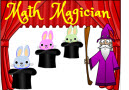# Math Magician GamesAre you a math wizard? Play these fun and interactive Math Magician games to show off your talents (such as speed and accuracy.) For each game, you can make 20 bunnies disappear by matching each math problem to the correct answer in just a few seconds.

It's not magic, it's MATH!

The games on this webpage are designed to provide students with a fun way to practice mental math and develop automaticity and fluency.

Math Magician Game (Addition, Subtraction, Multiplication) 2nd and 3rd grade students can practice adding, subtracting, and multiplying whole numbers by playing this fun game.

Math Magician Division Game
Can you quickly divide whole numbers? Who can make all 20 bunnies disappear? You have to be very fast when dragging and dropping each bunny into the correct magic hat.

Fun! Fun! Fun! That's what kids will be provided when they solve the addition problems in this exciting Math Magician Addition Game.

Math Magician Subtraction Game
The kids can have a great time as they solve the subtraction problems in this exciting Math Magician Subtraction Game.

Math Magician Compare Fractions Game
Spend your learning time in a fun way by tackling these comparing fractions problems with this exciting Math Magician Compare Fractions Game.

Math Magician Simplest Form Game
Match the fractions on the bunnies in this fun Math Magician Simplest Form Game with their simplest form on the hats.

Math Magician Add Three Numbers Game
Try this neat little Math Magician Add Three Numbers Game to help improve your addition skills while having fun.

Math Magician Add Two-Digit Numbers Game
Show how well you can add two-digit numbers by playing this fun Math Magician Add Two-Digit Numbers Game.

Math Magician Integers Game
Is the solution positive, negative, or zero? Play this fun Math Magician Integers Game.

Math Magician Multiplication Game
Show how well you can multiply numbers by playing this fun Math Magician Multiplication Game.

Math Magician Factors Game
Match the factors on the bunnies to the correct numbers on the hats in this exciting Math Magician Factors Game.

Math Magician Rounding Game
Show how well you can round numbers to tens and hundreds by playing this fun Math Magician Rounding Game.

Math Magician Multiples Game
Match the numbers with their multiples by playing this fun Math Magician Multiples Game.

Math Magician Equivalent Fractions Game
Match the equivalent fractions in this fun Math Magician Equivalent Fractions Game. Get as much practice as possible by playing this cool game.

Return from the Math Magician Games page to Math Play homepage.